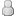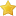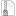Problem with pagination
leefishHamsterPosts: 1,009
Joined: Apr 2010
Post: #4
RE: Problem with pagination
I use frostschutz's patches plugin with the attached patch to fix this issue. This is what the code was prepatch:

PHP Code:
 `12345678910111213141516171819202122232425` ``````if(\$fpermissions['canviewthreads'] != 0) { // How many posts are there? if((\$datecut > 0 && \$datecut != 9999) || isset(\$fpermissions['canonlyviewownthreads']) && \$fpermissions['canonlyviewownthreads'] == 1) { \$query = \$db->simple_select("threads", "COUNT(tid) AS threads", "fid = '\$fid' \$useronly \$visibleonly \$datecutsql \$prefixsql"); \$threadcount = \$db->fetch_field(\$query, "threads"); } else { \$query = \$db->simple_select("forums", "threads, unapprovedthreads, deletedthreads", "fid = '{\$fid}'", array('limit' => 1)); \$forum_threads = \$db->fetch_array(\$query); \$threadcount = \$forum_threads['threads']; if(\$ismod == true) { \$threadcount += \$forum_threads['unapprovedthreads'] + \$forum_threads['deletedthreads']; } // If we have 0 threads double check there aren't any "moved" threads if(\$threadcount == 0) { \$query = \$db->simple_select("threads", "COUNT(tid) AS threads", "fid = '\$fid' \$useronly \$visibleonly", array('limit' => 1)); \$threadcount = \$db->fetch_field(\$query, "threads"); } } }``````

and post patch

PHP Code:
 `1234567891011121314151617181920212223242526` ``````if(\$fpermissions['canviewthreads'] != 0) { // How many posts are there? if(\$datecutsql || \$useronly || \$prefixsql) { \$query = \$db->simple_select('threads', 'COUNT(tid) AS threads', "fid='{\$fid}'{\$useronly}{\$visibleonly}{\$datecutsql}{\$prefixsql}", array('limit' => 1)); \$threadcount = \$db->fetch_field(\$query, "threads"); } else { \$query = \$db->simple_select("forums", "threads, unapprovedthreads, deletedthreads", "fid = '{\$fid}'", array('limit' => 1)); \$forum_threads = \$db->fetch_array(\$query); \$threadcount = \$forum_threads['threads']; if(\$ismod == true) { \$threadcount += \$forum_threads['unapprovedthreads'] + \$forum_threads['deletedthreads']; } // If we have 0 threads double check there aren't any "moved" threads if(\$threadcount == 0) { \$query = \$db->simple_select('threads', 'COUNT(tid) AS threads', "fid='{\$fid}'{\$visibleonly}", array('limit' => 1)); \$threadcount = \$db->fetch_field(\$query, "threads"); } } }``````

Attached File(s)patches.zip (Size: 1,013 bytes / Downloads: 381)